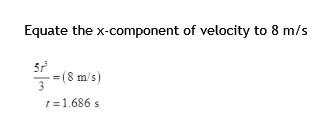A particle is at rest and at x=0 and y=0 at time = 0. The particle then moves in the x-y plane with an acceleration in m/s^2 of a = 5t^2 i + 5t j , where t is in seconds. Determine the magnitude of the particle's position vector in m at instant the x-component of the velocity vector vx = 8 m/s.

Question

A particle is at rest and at x=0 and y=0 at time = 0. The particle then moves in the x-y plane with an acceleration in m/s^2 of a = 5t^2 i + 5t j , where t is in seconds. Determine the magnitude of the particle's position vector in m at instant the x-component of the velocity vector vx = 8 m/s.

Step 1
Step 2help_outlineImage TranscriptioncloseEquate the x-component of velocity to 8 m/s (8 m/s) 1.686 s fullscreen
Step 3

Want to see the full answer?

See Solution

Want to see this answer and more?

Our solutions are written by experts, many with advanced degrees, and available 24/7

See Solution
Tagged in

Physics